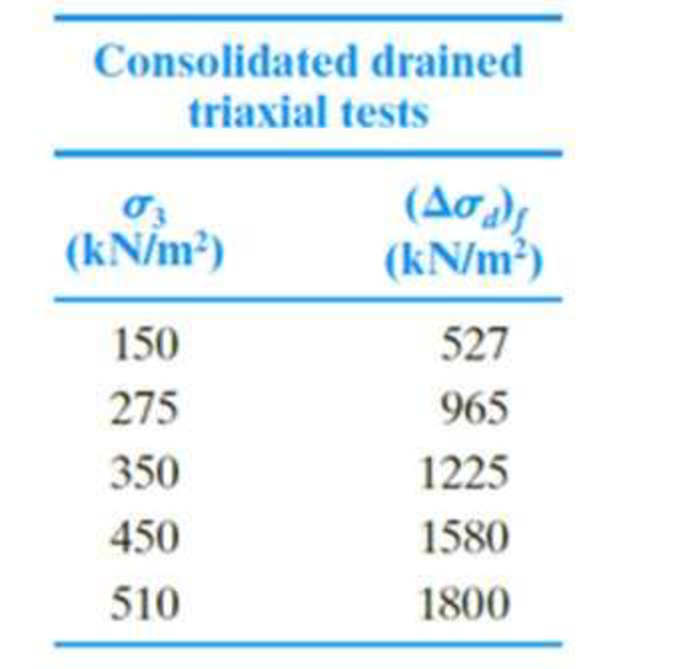Chapter 12, Problem 12.1CTPPrinciples of Geotechnical Enginee...

9th Edition
Braja M. Das + 1 other
ISBN: 9781305970939

Solutions

Chapter
SectionPrinciples of Geotechnical Enginee...

9th Edition
Braja M. Das + 1 other
ISBN: 9781305970939
Textbook Problem

In this chapter, you learned different types of triaxial tests. During a triaxial test, the successive stress states experienced by the soil specimen can be represented by diagrams called stress paths. Schofield and Wroth (1968) defined the stress states in terms of p′ (mean normal effective stress) and q (deviator stress) as follows: p ′ = 1 3 ( σ ′ 1 + σ ′ 2 + σ ′ 3 ) = 1 3 ( σ ′ 1 + 2 σ ′ 3 ) (Note: σ ′ 2 = σ ′ 3 for triaxial tests) q = σ 1 − σ 3 A stress path is a line joining each progressive stress state plotted on a p′–q graph, as shown in Figure 12.56. A soil element in the field may go through various complicated stress paths during the lifetime of a geotechnical structure. It is sometimes possible to simulate the field conditions using advanced triaxial stress path testing, in which the axial and confining pressures are independently controlled and varied to achieve a desired stress path in the p′–q space. This way. soil behavior can be predicted under more realistic field loading conditions. In this problem, we will learn how stress paths are constructed for consolidated drained triaxial tests.Task 1: Establishing the Failure Line ill the p′–q SpaceThe following table shows the results of a series of consolidated drained triaxial tests on a medium dense granular soil. Draw the stress paths for each test in the p′–q space. Also, establish the failure line going through the origin and connecting the failure points ( p ′ f , q f ) .Task 2: Loading the Specimen Through a Specified Stress PathA soil specimen is loaded along the stress path O (0,0), P (250,0), and A (675, 1000) under drained conditions to reach point A close to the failure line established in Task 1 (Figure 12.56). Determine the combinations of confining pressure and deviator stresses applied to the triaxial specimen at O, P, and A in order to follow the stress path O-P-A.To determine

Draw the stress paths for each test in the pq space and also establish the failure line going through the origin and connecting the failure points.

Explanation

Find the major effective principal stress (σ1) as shown below:

σ1=σ3+(Δσd)f (1)

Here, σ3 is chamber pressure and (Δσd)f is deviator stress at failure.

Substitute 150kN/m2 for σ3, 527kN/m2 for (Δσd)f in Equation (1).

σ1=527+150=677kN/m2

Find the effective stress at failure (pf) using the relation:

pf=13(σ1+2σ3) (2)

Here, σ3 and σ1 minor and major effective principal stress at failure.

Substitute 677kN/m2 for σ1 and 150kN/m2 for σ3 in Equation (2).

pf=13(677+2(150))=13×977=325

To determine

Find the combinations of confining pressure and deviator stress applied to the triaxial specimen at O, P, and A in order to follows the stress path O-P-A.

Still sussing out bartleby?

Check out a sample textbook solution.

See a sample solution

The Solution to Your Study Problems

Bartleby provides explanations to thousands of textbook problems written by our experts, many with advanced degrees!

Get Started

At what angle will the lamp in Prob. 4.16 be in equilibrium without the couple CA?

International Edition---engineering Mechanics: Statics, 4th Edition

What are data warehousing and data mining? How do businesses use these tools?

Systems Analysis and Design (Shelly Cashman Series) (MindTap Course List)

Use an elastic analysis and determine the maximum load in the weld (in kips per inch of length).

Steel Design (Activate Learning with these NEW titles from Engineering!)

How does an uplink differ from a downlink?

Enhanced Discovering Computers 2017 (Shelly Cashman Series) (MindTap Course List)

Name three popular welding processes.

Welding: Principles and Applications (MindTap Course List)